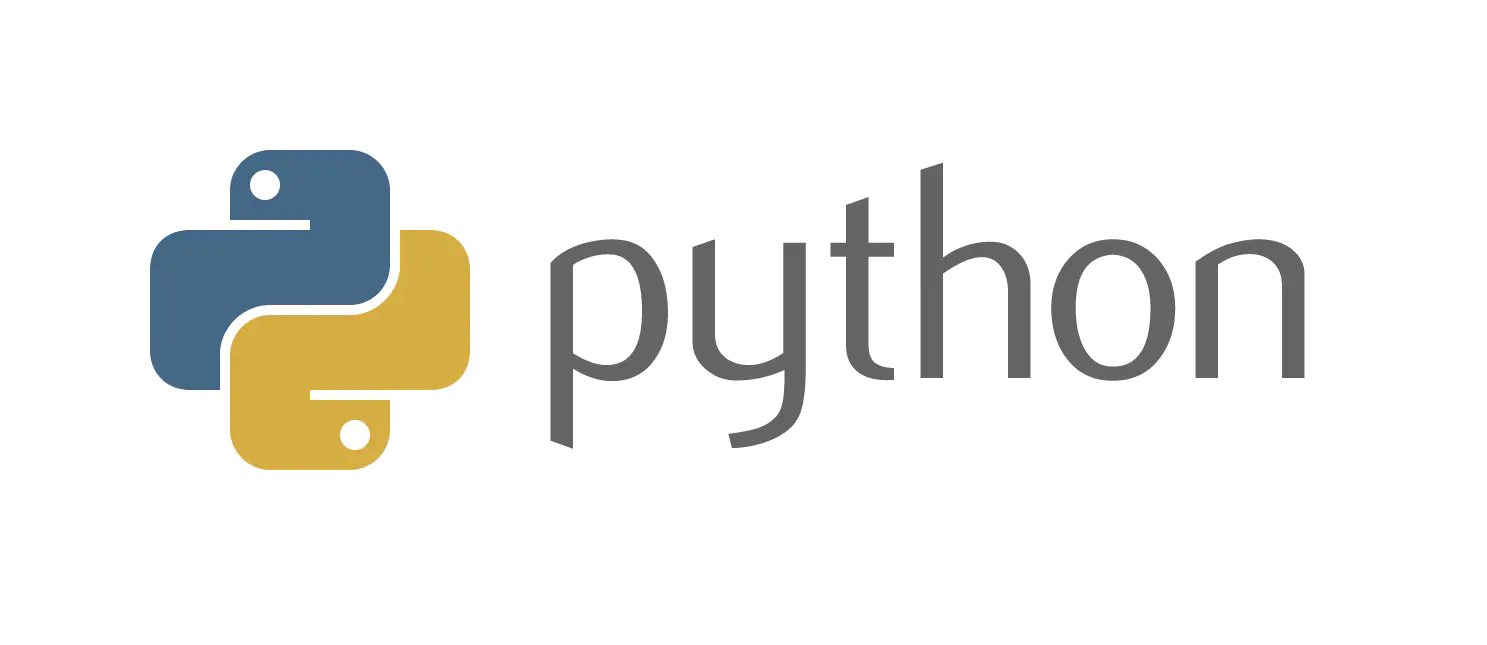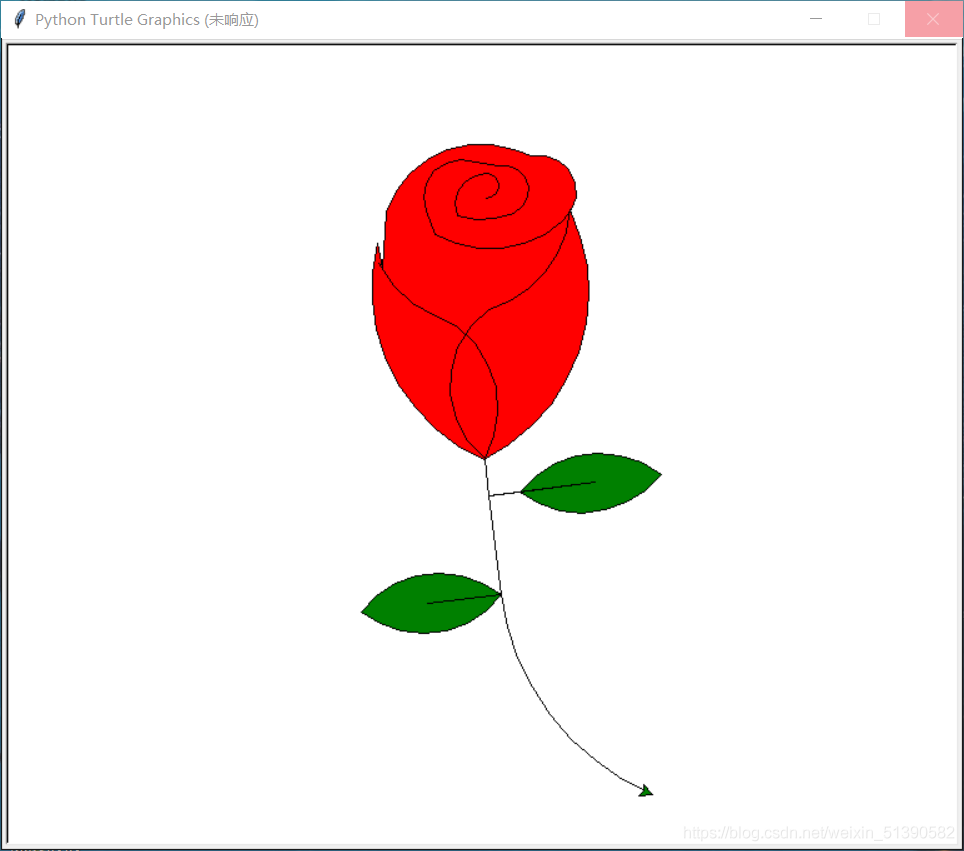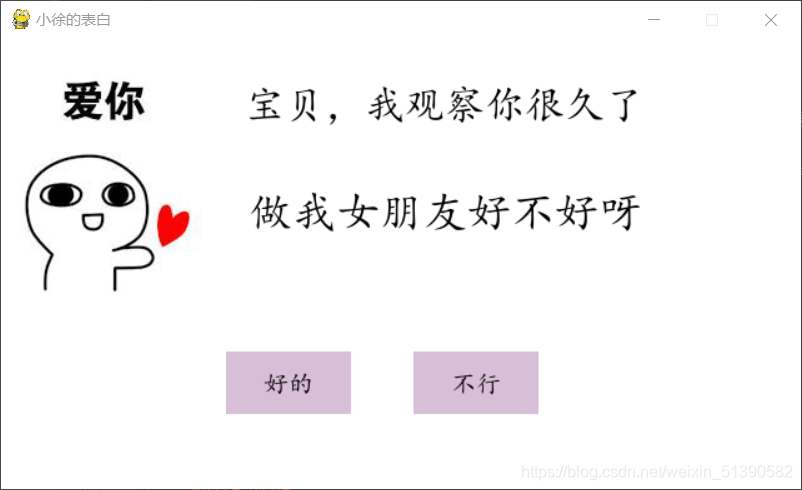# ❤️Python入门这些你必须要知道！语言简介+语法基础+应用实战 熬夜整理，建议收藏~❤️

## Python入门这些你必须要知道！📢📢📢📣📣📣
🌻🌻🌻Hello，大家好我叫是Dream呀，一个有趣的Python博主，小白一枚，多多关照😜😜😜
🏅🏅🏅CSDN Python领域新星创作者，作者周榜NO.23 ，大二在读
💕入门须知：这片乐园从不缺乏天才，努力才是你的最终入场券！🚀🚀🚀
💓最后，愿我们都能在看不到的地方闪闪发光，一起加油进步🍺🍺🍺
🍉🍉🍉“一万次悲伤，依然会有Dream，我一直在最温暖的地方等你”，唱的就是我！哈哈哈~🌈🌈🌈
🌟🌟🌟✨✨✨

### PYTHON

hello，大家好，我是Dream，今天给大家介绍一下Python和Python的一些基础知识及应用！先点赞收藏起来吧~!# Python方向简介及前言

Python第一个公开发行版发行于1991年，所以这年被当作Python的诞生年。
Python 源代码遵循 GPL(GNU General Public License)协议，这是一个开源的协议，也就是说你可以免费使用和传播它，而不用担心版权的问题。# Python语言特点

1. 简单易学、明确优雅、开发速度快
✍简单易学：与C和Java比，Python的学习成本和难度曲线不是低一点，更适合新手入门，自底向上的技术攀爬路线。
✍明确优雅：Python的语法非常简洁，代码量少，非常容易编写，代码的测试、重构、维护等都非常容易。
✍开发速度快：当前互联网企业的生命线是什么？产品开发速度！如果你的开发速度不够快，在你的产品推出之前别人家的产品已经上线了，你也就没有生存空间了，这里的真实例子数不胜数。那么，Python的开发速度说第二没人敢称第一!
2. 跨平台、交互式、解释型、面向对象的动态语言
✍跨平台：Python支持Windows、Linux和MAC os等主流操作系统。
✍交互式：Python提供很好的人机交互界面，比如IDLE和IPython。可以从终端输入执行代码并获得结果，互动的测试和调试代码片断。
✍解释型：Python语言在执行过程中由解释器逐行分析，逐行运行并输出结果。
✍面向对象：Python语言具备所有的面向对象特性和功能，支持基于类的程序开发。
✍动态语言：在运行时可以改变其结构。例如新的函数、对象、甚至代码可以被引进，已有的函数可以被删除或是其他结构上的变化。动态语言非常具有活力。
3.大量的标准库和第三方库
✍Python为我们提供了非常完善的基础库，覆盖了系统、网络、文件、GUI、数据库、文本处理等方方面面，这些是随同解释器被默认安装的，各平台通用，你无需安装第三方支持就可以完成大多数工作。
4. 开源语言，发展潜力巨大
✍Python是基于C语言编写的，并且使用GPL开源协议，你可以免费获取它的源代码，进行学习、研究甚至改进。众人拾柴火焰高，有更多的人参与Python的开发，促使它更好的发展，被更多的应用，形成良性循环。
✍Python为什么会越来越火就是因为它的开放性，自由性，聚起了人气，形成了社区，有很多人在其中做贡献，用的人越来越多，自然就提高了市场占有率，企业、公司、厂家就不得不使用Python，提供的Python程序员岗位就越来越多，这就是开源的力量。

# Python可以做什么AI

WEB开发

## Python之禅

The Zen of Python, by Tim Peters
Beautiful is better than ugly.
Explicit is better than implicit.
Simple is better than complex.
Complex is better than complicated.
Flat is better than nested.
Sparse is better than dense.
Special cases aren’t special enough to break the rules.
Although practicality beats purity.
Errors should never pass silently.
Unless explicitly silenced.
In the face of ambiguity, refuse the temptation to guess.
There should be one-- and preferably only one --obvious way to do it.
Although that way may not be obvious at first unless you’re Dutch.
Now is better than never.
Although never is often better than right now.
If the implementation is hard to explain, it’s a bad idea.
If the implementation is easy to explain, it may be a good idea.
Namespaces are one honking great idea – let’s do more of those!

# Python基础知识及应用

## 语法基础：

#将字符列表转化为字符串
l=['Python','Circle','is','ok']
j=''.join(l)
print(j)

#将字符串居中
a='徐以鹏比易烊千玺帅亿点'
b=a.center(50)
c=a.center(50,'*')
print(b)
print(c)

#find方法找到字符串中的字符，找到就返回第一个字符的索引，找不到就返回-1
a='I love python'
b=a.find('o')
print(b)
c=a.find('lov')
print(c)

#让字符的首字母大写，其他字母手写
a='i love python'
b=a.title()
c='I LOVE PYTHON'
d=c.title()
print(b)
print(d)

#用string中的capwords方法
import string
a='i lOve python'
b=string.capwords(a)
print(b)

#清空列表
a=[1,2,3]
a.clear()
print(a)
a=[1,2,3]
a[:]=[]
print(a)

#计算指定元素在列表中出现的次数
a=['i','am','ok','k','ok']
b=a.count('ok')
print(b)

#在列表末尾加入元素
a=[1,2,3]
b=[4,5,6]
c=a+b
print(c)
a=[1,2,3]
b=[4,5,6]
a.extend(b)
print(a)

#将对象插入到列表中
a=[1,2,3,4]
a.insert(2,'there')
print(a)
a=[1,2,3,4]
a[2:2]=['there']
print(a)

#删除列表中的元素
a=[1,2,3,4]
a.pop()#pop可以删除指定元素，默认删除最后一个元素
print(a)
a.pop(0)
print(a)

#删除列表中的指定元素
a=[1,2,4,3,4]
a.remove(4)#只删除第一次出现的元素
print(a)

#让列表按相反顺序排序？
a=[2,1,5,99]
a.reverse()
print(a)
a=[2,1,5,99]
a=a[::-1]
print(a)

#表示只包含一个元素的元组，一定要在后面加逗号
a=(1)
print(type(a))#整数
a=(1,)
print(type(a))#元组

#批量替换字符串中的元素
a='i love python'
b=a.replace('o','徐')
print(b)

#将字符串按照空格进行拆分
a='i love python'
print(a.split())

#去除字符串首位的空格
a=' i love python'
print(a.strip())

#给字典中不存在的key指定默认值
a={'age':42,'name':'g'}
print(a.get('aa','N/2'))

#快速求1到100所有整数相加的和
print(sum(range(1,101)))

'''#快速启动浏览器打开指定的网站，使用webbrowser库
import webbrowser
webbrowser.open('http://www.python.org')'''

#给函数编写文档在 def 语句后⾯把注释⽂档放在引号（单引、双引、三引都可以）⾥⾯就⾏，这个⽂档可以通过 function.__doc__访问。
def square(x):
'''返回平方值'''
return x*x
print(square.__doc__)

#定义私有方法
#在方式名称前加两个下斜杠
class Person:
def __name(self):
print('私有方法')
#注明：⽤ from module import * 导⼊时不会导⼊私有⽅法。

#怎么判断一个类是另一个类的子类？
class A:
pass
class B(A):
pass
print(issubclass(A,B))#是返回True，不是返回False

#怎么从一个非空序列中随机选择一个元素？
import random
print(random.choice([1,'two',3,'四']))

#怎么查出通过from xx import xx导入的可以直接调用的方法？
#⽤ all ⽅法，这个⽅法查出的是模块下不带_的所有⽅法，可以直接调⽤。
import random
print(random.__all__)

#花括号{}是集合还是字典：是字典

#怎么求两个集合的并集？
a={6,7,8}
b={7,8,9}
print(a.union(b))

a={6,7,8}
b={7,8,9}
print(a|b)

#求两个集合的交集
a={6,7,8}
b={7,8,9}
print(a&b)

a={6,7,8}
b={7,8,9}
print(a.intersection(b))

#求两个集合中不重复的元素
a={6,7,8}
b={7,8,9}
print(a^b)

a={6,7,8}
b={7,8,9}
print(a.symmetric_difference(b))

#求两个集合的差集
a={6,7,8}
b={7,8,9}
print(a-b)

a={6,7,8}
b={7,8,9}
print(b.difference(a))
print(a.difference(b))

#从一个序列中随机返回n个不同值的元素
import random
t=(2020,7,3,21,48,56,4,21,0)
print(random.sample(t,2))

#怎么生成两个数之间的随机实数
from random import uniform
print(uniform(10,20))
import random
print(random.uniform(10,20))

#怎么在等差数列中随机选择一个数
print(random.randrange(0,100,10))

#怎么在文件中写入字符？
'''#使用open函数，模式用w
with open('bruce.txt','w')as f:
f.write('hello word')'''

with open('bruce.txt','r')as f:

#怎样将当前时间转化为字符串？
#用time模块中的asctime方法
import time
print(time.asctime())

#怎么随机打乱列表的顺序
import random
t=list(range(20))
print(t)
random.shuffle(t)
print(t)



## 高级语法：

#用for循环实现把字符串变成Unicode码未的列表:
a='!@#$%^&*(' codes=[] for i in a: codes.append(ord(i)) print(codes) #怎样用列表推导式把字符串变成Unicode码位的列表 a="!@#$"
codes=[ord(s) for s in a]
print(codes)
#很明显，⽤列表推导式实现⽐ for 循环加 append 更⾼效简洁，可读性更好。

#打印出两个列表的笛卡尔积
#解法1:使⽤⽣成器表达式产⽣笛卡尔积，可以帮忙省掉运⾏ for 循环的开销。
colors=['black','white']
sizes=['S','M','l']
for tshirts in ('%s %s'%(c,s)for c in colors for s in sizes):
print(tshirts)
#解法2:使⽤ itertools ⾥的 product ⽣成器函数。
import itertools
print(list(itertools.product(['black','white'],['S','M','L'])))

#用神魔方法接收不确定值或者参数
#⽤ *args 的⽅式，*args 位置可以在任意位置。
a,b,*c=range(8)
print(a,b,c)
a,*b,c,d=range(5)
print(a,b,c,d)
*a,b,c,d=range(5)
print(a,b,c,d)

#sort与sorted的区别
l=[1,9,5,8]
l.sort()
print(l)
print(sorted(l))
#sort() 会就地在原序列上排序，sorted() 新建了⼀个新的序列。

#怎么通过 reverse 参数对序列进⾏降序排列
l=[1,9,5,8]
j=sorted(l,reverse=True)
print(j)

#快速插⼊元素到列表头部
#1.切片
l=[1,2,3,4,5]
l[0:0]='Python'
print(l)
#将python分开了
#2.用insert方法
l=[1,2,3,4,5]
l.insert(0,'first')
print(l)

#创建字典
a = dict(one=1, two=2, three=3)
b = {'one': 1, 'two': 2, 'three': 3}
c = dict(zip(['one', 'two', 'three'], [1, 2, 3]))
d = dict([('two', 2), ('one', 1), ('three', 3)])
e = dict({'one': 1, 'two': 2, 'three': 3})
print(a,b,c,d,e)

#列表去重
l=['A','A','B','B']
print(list(set(l)))

#Python中怎么定义私有属性。
#在属性前加两个前导下划线，尾部没有或最多有⼀个下划线

#怎么随机打乱⼀个列表⾥元素的顺序
from random import shuffle
l=list(range(30))
shuffle(l)
print(l)

#进度条显示
import time
from tqdm import tqdm
for i in tqdm(range(1000)):
time.sleep(.01)



## Python中的Pygame模块：

Pygame是跨平台Python模块，专为电子游戏设计，包含图像、声音。建立在SDL基础上，允许实时电子游戏研发而无需被低级语言（如机器语言和汇编语言）束缚。

pip install pygame

Python制作打砖块游戏，思路清晰，好玩又不失新颖，值得一试！## Python海龟画图import turtle
import time

# 设置初始位置
turtle.penup()
turtle.left(90)
turtle.fd(200)
turtle.pendown()
turtle.right(90)

# 花蕊
turtle.fillcolor("red")
turtle.begin_fill()
turtle.circle(10, 180)
turtle.circle(25, 110)
turtle.left(50)
turtle.circle(60, 45)
turtle.circle(20, 170)
turtle.right(24)
turtle.fd(30)
turtle.left(10)
turtle.circle(30, 110)
turtle.fd(20)
turtle.left(40)
turtle.circle(90, 70)
turtle.circle(30, 150)
turtle.right(30)
turtle.fd(15)
turtle.circle(80, 90)
turtle.left(15)
turtle.fd(45)
turtle.right(165)
turtle.fd(20)
turtle.left(155)
turtle.circle(150, 80)
turtle.left(50)
turtle.circle(150, 90)
turtle.end_fill()

# 花瓣1
turtle.left(150)
turtle.circle(-90, 70)
turtle.left(20)
turtle.circle(75, 105)
turtle.circle(80, 98)
turtle.circle(-90, 40)

# 花瓣2
turtle.left(180)
turtle.circle(90, 40)
turtle.circle(-80, 98)

# 叶子1
turtle.fd(30)
turtle.left(90)
turtle.fd(25)
turtle.left(45)
turtle.fillcolor("green")
turtle.begin_fill()
turtle.circle(-80, 90)
turtle.right(90)
turtle.circle(-80, 90)
turtle.end_fill()

turtle.right(135)
turtle.fd(60)
turtle.left(180)
turtle.fd(85)
turtle.left(90)
turtle.fd(80)

# 叶子2
turtle.right(90)
turtle.right(45)
turtle.fillcolor("green")
turtle.begin_fill()
turtle.circle(80, 90)
turtle.left(90)
turtle.circle(80, 90)
turtle.end_fill()

turtle.left(135)
turtle.fd(60)
turtle.left(180)
turtle.fd(60)
turtle.right(90)
turtle.circle(200, 60)
time.sleep(10)



## 综合应用

Python表白神器，源码+解析+各种完美配置+浪漫新颖# 最后的福利

09-17201
12-192079

03-18313
10-2946
06-152万+
06-17194
12-17127
10-268946
05-22281
09-27108
09-071939
08-263183
10-31163
05-1365
12-1226
11-291368
08-052173
08-29178
09-126212

### “相关推荐”对你有帮助么？

•非常没帮助
•没帮助
•一般
•有帮助
•非常有帮助¥2 ¥4 ¥6 ¥10 ¥20余额支付 (余额：-- )扫码支付获取中扫码支付点击重新获取扫码支付1.余额是钱包充值的虚拟货币，按照1:1的比例进行支付金额的抵扣。
2.余额无法直接购买下载，可以购买VIP、C币套餐、付费专栏及课程。余额充值